## Week 1 Lecture 3: Matrix Syntax

If you are familiar with linear algebra or physics, then you already know the importance of matrices. If you have not encountered matrices before, you can think of them as several row vectors stacked on top of each other or as several column vectors side by side. For instance, the following matrix can either be seen as four row vectors, each with three entries, or as three column vectors, each with four entries. We call it a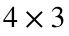matrix.In general, an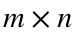matrix has m rows and n columns.

### Constructing matrices

MATLAB has very convenient syntax for constructing matrices. It looks essentially the same as that for both vector types at once. You surround the entire matrix with square brackets and type entries from left to right, top to bottom. Entries in the same row can be separated with spaces or commas and different rows are separated by semicolons. For example, the above matrix can be defined in MATLAB with
A = [-1 2 1; 3 0 1; 4 -2 2; -2 1 3]
A = 4×3
-1 2 1 3 0 1 4 -2 2 -2 1 3
Similarly, we can make a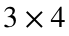matrix B and two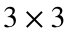matrices C and D:
B = [1 -2 0 3; 2 1 -4 1; -3 0 1 1]
B = 3×4
1 -2 0 3 2 1 -4 1 -3 0 1 1
C = [1 0 2; 3 -1 1; 2 2 0]
C = 3×3
1 0 2 3 -1 1 2 2 0
D = [1 2 -1; 3 -2 2; -1 1 0]
D = 3×3
1 2 -1 3 -2 2 -1 1 0
It is not a coincidence that this syntax looks so similar to that for vectors. MATLAB treats vectors as special cases of matrices. In particular, a row vector with n entries is exactly the same as a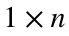matrix and a column vector with m entries is exactly the same as an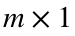matrix. In fact, MATLAB treats every variable as a matrix. If a variable only has one number, then it is just a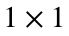matrix.

### Matrix arithmetic

Since matrices and vectors are so similar in MATLAB, it should not surprise you that the basic arithmetic operations work the same with matrices as they do with vectors. In particular, you can add or subtract the same number from each entry of a matrix and multiply or divide every entry in a matrix by the same number. For example:
A + 5
ans = 4×3
4 7 6 8 5 6 9 3 7 3 6 8
B - 2
ans = 3×4
-1 -4 -2 1 0 -1 -6 -1 -5 -2 -1 -1
C * 3
ans = 3×3
3 0 6 9 -3 3 6 6 0
D / 4
ans = 3×3
0.2500 0.5000 -0.2500 0.7500 -0.5000 0.5000 -0.2500 0.2500 0
Furthermore, you can add or subtract two matrices of exactly the same size and shape with the + and - operators. This will add/subtract corresponding entries of the matrices. So, for instance,
C + D
ans = 3×3
2 2 1 6 -3 3 1 3 0
C - D
ans = 3×3
0 -2 3 0 1 -1 3 1 0
As with vectors, adding/subtracting matrices of different sizes/shapes will either give an error or produce unexpected results. You should check what happens in the command window when you try
A + B
or
C - A
As with vectors, you cannot generally multiply or divide two matrices (and when it does work it will not multiply/divide entry by entry like you might expect). If you want to multiply/divide each element of one matrix with the corresponding elements of another matrix, you can use .* and ./. For instance,
C .* D
ans = 3×3
1 0 -2 9 2 2 -2 2 0
C ./ D
ans = 3×3
1.0000 0 -2.0000 1.0000 0.5000 0.5000 -2.0000 2.0000 NaN
As before, this only works when the matrices are exactly the same size/shape.

### Accessing and modifying matrices

Accessing and modifying entries of a matrix works much like it did with vectors. Recall that you can specify an entry of a vector by giving a whole number index, so x(3) means "the third entry of x". You can do the same thing with matrices, but MATLAB counts indices in a way you might not expect.
For instance, if we redefine
A = [-1 2 1; 3 0 1; 4 -2 2; -2 1 3]
A = 4×3
-1 2 1 3 0 1 4 -2 2 -2 1 3
then we can try
A(3)
ans = 4
A(4)
ans = -2
A(5)
ans = 2
A(11)
ans = 2
Notice that MATLAB counts from top to bottom, then from left to right. That is, when you use this syntax MATLAB pretends that the matrix A is three columns stacked on top of each other in a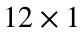column vector. (In fact, that is how MATLAB stores matrices internally. We won't need to worry about this fact, but if you are trying to make your MATLAB code as fast as possible then it becomes quite important.) This is not very convenient syntax to work with, so we generally use a slightly different format. Instead of just specifying one index, we specify both a row and column index. The general syntax is matrix_name(row_index, column_index). For example, to read the number in the third row, second column of A, we can type
A(3, 2)
ans = -2
Likewise, if we wanted to set the entry in the fourth row, first column to 100, we could write
A(4, 1) = 100
A = 4×3
-1 2 1 3 0 1 4 -2 2 100 1 3
All of the same shortcuts we have learned for vector indices apply to both the row indices and column indices of a matrix. For instance, you can use end to refer to the last row or column:
A(2, end)
ans = 1
A(end, 2)
ans = 1
A(end - 1, end)
ans = 2
A(end, end)
ans = 3
You can also use the colon operator to specify several rows or columns at once:
A(2:3, 1:3)
ans = 2×3
3 0 1 4 -2 2
A(1:2:end, 2)
ans = 2×1
2 -2
You can also modify multiple elements at once. As with vectors, you can set all the chosen elements to a single value like this:
A(2:3, 2:3) = 8
A = 4×3
-1 2 1 3 8 8 4 8 8 100 1 3
or you can specify the new value of every element you want to change:
A(2:3, 2:3) = [1 2; 3 4]
A = 4×3
-1 2 1 3 1 2 4 3 4 100 1 3
In the latter case, the matrix on the right of the = operator must be exactly the same size/shape as the block you are changing.
There is one other very useful shortcut for matrices. If you just use the colon operator : without any other numbers, it means "all possible indices". For example,
A(:, 2)
ans = 4×1
2 1 3 1
prints every row of the second column. Likewise,
B(1:2, :)
ans = 2×4
1 -2 0 3 2 1 -4 1
prints every column of the first and second rows. This syntax actually works for vectors as well, but it isn't particularly useful. x(:) or y(:) print every entry of a vector x or y. For somewhat technical reasons, this syntax always produces a column vector.

### Matrix multiplication

If you do some experimentation, you will find that you can sometimes use the * operator with two matrices, even if they are not the same dimensions. We are now in a position to talk about what expressions like A * B mean. First, we need to review the concept of the dot product. The dot product of two vectors of the same length is the sum of the products of their corresponding entries. For example, ifand,
then the dot product of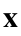and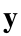(which we denote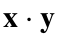) is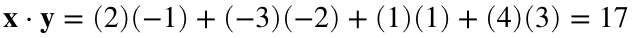.
We can calculate this in MATLAB with
x = [2; -3; 1; 4];
y = [-1; -2; 1; 3];
x(1)*y(1) + x(2)*y(2) + x(3)*y(3) + x(4)*y(4)
ans = 17
This is a common enough operation that MATLAB has a builtin command for computing the dot product. It is named, appropriately enough, dot. You can use it like this:
dot(x, y)
ans = 17
In general, ifandare vectors of length n, then we define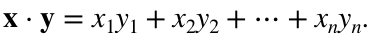Note that this definition only makes sense whenandare the same length.
We will define matrix multiplication as follows: If A is anmatrix and B is an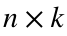matrix, then the product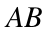is an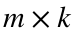matrix where the entry in the ith row and jth column is the dot product of the ith row of A with the jth column of B. In MATLAB syntax, this means that
AB(i, j) = dot(A(i, :), B(:, j))
It is important to notice that this definition only works when the number of columns of A is the same as the number of rows of B, because that way we will only take dot products of vectors of the same length.
This definition may seem overly complicated, but it turns out to be very useful. We will go into much more detail about why when we start solving linear systems. For now, you just need to remember that MATLAB does this version of multiplication when you use the * symbol. For instance,
A = [-1 2 1; 3 0 1; 4 -2 2; -2 1 3];
B = [1 -2; 2 1; -3 0];
A and B areand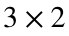matrices, respectively. Since the number of columns of A is the same as the number of rows of B, we can multiply A times B. In MATLAB, this is just
A * B
ans = 4×2
0 4 0 -6 -6 -10 -9 5
However, the number of columns of B is not the same as the number of rows of A, so we cannot multiply B times A. If you try the code
B * A
you will get an error. This also explains why we couldn't use the syntax x * x when x was a vector. Unless x only had one entry, the number of rows wouldn't be the same as the number of columns, so we couldn't use this definition.
From now on, whenever we talk about matrix multiplication in this class, we will mean this definition. That is, if you see an expressionor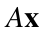, you can use the * operator in MATLAB.

### MATLAB documentation

We introduced our first function in this lecture ( dot), so now is a good time to talk about MATLAB documentation. MATLAB has extensive help files for all of its builtin functions. If you know the name of a function but aren't sure exactly how to use it, the first thing you should do is go to the command window and type doc function_name. For instance, if you want to look up the dot function, you can use
doc dot
This will pop up a window with lots of information about the function. (If, for some reason, you don't want to pop out a window, you can also use the command help.
help dot
dot Vector dot product. C = dot(A,B) returns the scalar product of the vectors A and B. A and B must be vectors of the same length. When A and B are both column vectors, dot(A,B) is the same as A'*B. dot(A,B), for N-D arrays A and B, returns the scalar product along the first non-singleton dimension of A and B. A and B must have the same size. dot(A,B,DIM) returns the scalar product of A and B in the dimension DIM. Class support for inputs A,B: float: double, single See also cross. Documentation for dot Other functions named dot
This just provides a text version of the same information.) I highly recommend that you use doc whenever you encounter a new function. Even if you're pretty sure you know how a function works, it never hurts to quickly check the documentation and make sure. If you don't remember the name of a function, you can often find it just by using the search bar in the documentation window.